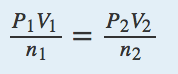Chapter 6. Gases

# End-of-Chapter Material

David W. Ball

### Additional Exercises

1. What is the pressure in pascals if a force of 4.88 kN is pressed against an area of 235 cm2?
2. What is the pressure in pascals if a force of 3.44 ×104 MN is pressed against an area of 1.09 km2?
3. What is the final temperature of a gas whose initial conditions are 667 mL, 822 torr, and 67°C, and whose final volume and pressure are 1.334 L and 2.98 atm, respectively? Assume the amount remains constant.
4. What is the final pressure of a gas whose initial conditions are 1.407 L, 2.06 atm, and −67°C, and whose final volume and temperature are 608 mL and 449 K, respectively? Assume the amount remains constant.
5. Propose a combined gas law that relates volume, pressure, and amount at constant temperature.
6. Propose a combined gas law that relates amount, pressure, and temperature at constant volume.
7. A sample of 6.022 ×1023 particles of gas has a volume of 22.4 L at 0°C and a pressure of 1.000 atm. Although it may seem silly to contemplate, what volume would one particle of gas occupy?
8. One mole of liquid N2 has a volume of 34.65 mL at −196°C. At that temperature, 1 mol of N2 gas has a volume of 6.318 L if the pressure is 1.000 atm. What pressure is needed to compress the N2 gas to 34.65 mL?
9. Use two values of R to determine the ratio between an atmosphere and a torr. Does the number make sense?
10. Use two values of R to determine how many joules are in a liter·atmosphere.

11.  At an altitude of 40 km above the earth’s surface, the atmospheric pressure is 5.00 torr, and the surrounding temperature is −20°C. If a weather balloon is filled with 1.000 mol of He at 760 torr and 22°C, what is its

a)  initial volume before ascent?

b)  final volume when it reaches 40 km in altitude? (Assume the pressure of the gas equals the surrounding pressure.)

12.  If a balloon is filled with 1.000 mol of He at 760 torr and 22°C, what is its

a)  initial volume before ascent?

b)  final volume if it descends to the bottom of the Mariana Trench, where the surrounding temperature is 1.4°C and the pressure is 1,060 atm?

13.  Air, a mixture of mostly N2 and O2, can be approximated as having a molar mass of 28.8 g/mol. What is the density of air at 1.00 atm and 22°C? (This is approximately sea level.)

14.  Air, a mixture of mostly N2 and O2, can be approximated as having a molar mass of 28.8 g/mol. What is the density of air at 0.26 atm and −26°C? (This is approximately the atmospheric condition at the summit of Mount Everest.)

15.  On the surface of Venus, the atmospheric pressure is 91.8 atm, and the temperature is 460°C. What is the density of CO2 under these conditions? (The Venusian atmosphere is composed largely of CO2.)

16.  On the surface of Mars, the atmospheric pressure is 4.50 torr, and the temperature is −87°C. What is the density of CO2 under these conditions? (The Martian atmosphere, similar to its Venusian counterpart, is composed largely of CO2.)

17.  HNO3 reacts with iron metal according to Fe(s) + 2HNO3(aq) → Fe(NO3)2(aq) + H2(g) In a reaction vessel, 23.8 g of Fe are reacted but only 446 mL of H2 are collected over water at 25°C and a pressure of 733 torr. What is the percent yield of the reaction?

18.  NaHCO3 is decomposed by heat according to 2NaHCO3(s) → Na2CO3(s) + H2O(ℓ) + CO2(g) If you start with 100.0 g of NaHCO3 and collect 10.06 L of CO2 over water at 20°C and 0.977 atm, what is the percent yield of the decomposition reaction?

19.  Determine if the following actions will cause the pressure of a particular gas sample to increase, decrease, or remain the same:

a)  decreasing the temperature

b)  decreasing the molar mass of the gas

c)  decreasing the volume of the container

20.  Under what conditions do gases deviate most from ideal gas behaviour? Explain your answer.

21.  Place the following gases in order from lowest to highest average molecular speed at 25°C: He, Ar, O2, I2.

22.  The effusion rate of an unknown noble gas sample is 0.35 times that of neon, at the same temperature. Determine the molecular weight and identity of the unknown noble gas.

23.  Use the van der Waal’s equation to determine the pressure of 2.00 moles of helium in a 5.00 L balloon at 300.00 K. How does this value compare to what you would obtain with the ideal gas law?

### Answers

1. 208,000 Pa

3. 1,874 K

5.7. 3.72 × 10-23 L

9. 1 atm = 760 torr

11.

11.1. 24.2 L
11.2. 3155 L

13. 1.19 g/L

15. 67.2 g/L

17. 3.99%

19.

19.1. decreased pressure
19.2. increased pressure
19.3. increased pressure

21. I< Ar < O< He

23. van der Waals: 9.94 atm, ideal: 9.85 atm

## LicenseEnd-of-Chapter Material by David W. Ball is licensed under a Creative Commons Attribution-NonCommercial-ShareAlike 4.0 International License, except where otherwise noted.